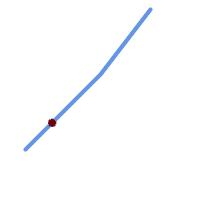## Name

ST_LineInterpolatePoint — 返回沿线在百分比指示位置的插值点。

## Synopsis

`geometry ST_LineInterpolatePoint(`geometry a_linestring, float8 a_fraction`)`;

`geography ST_LineInterpolatePoint(`geography a_linestring, float8 a_fraction, boolean use_spheroid = true`)`;

## 描述此函数计算 2D 中的点，然后对 Z 和 M 的值进行插值，而 ST_3DLineInterpolatePoint 计算 3D 中的点，然后仅对 M 值进行插值。从版本 1.1.1 开始，此函数还会插入 M 和 Z 值（如果存在），而之前的版本将它们设置为 0.0。该函数支持 3d 并且不会丢失 z-index。

## 示例```-- The point 20% along a line

SELECT ST_AsEWKT(  ST_LineInterpolatePoint(
'LINESTRING(25 50, 100 125, 150 190)',
0.2 ));
----------------
POINT(51.5974135047432 76.5974135047432)
```

3D 线的中点：

```SELECT ST_AsEWKT(  ST_LineInterpolatePoint('
LINESTRING(1 2 3, 4 5 6, 6 7 8)',
0.5 ));
--------------------
POINT(3.5 4.5 5.5)
```

```SELECT ST_AsText( ST_LineInterpolatePoint( line.geom,
ST_LineLocatePoint( line.geom, 'POINT(4 3)')))
FROM (SELECT ST_GeomFromText('LINESTRING(1 2, 4 5, 6 7)') As geom) AS line;
------------
POINT(3 4)
```